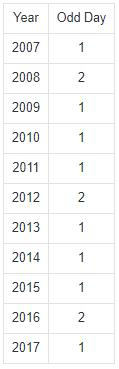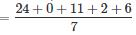Courses

# Test: Calendar 1

## 20 Questions MCQ Test UPSC Prelims Paper 2 CSAT - Quant, Verbal & Decision Making | Test: Calendar 1

Description
This mock test of Test: Calendar 1 for Quant helps you for every Quant entrance exam. This contains 20 Multiple Choice Questions for Quant Test: Calendar 1 (mcq) to study with solutions a complete question bank. The solved questions answers in this Test: Calendar 1 quiz give you a good mix of easy questions and tough questions. Quant students definitely take this Test: Calendar 1 exercise for a better result in the exam. You can find other Test: Calendar 1 extra questions, long questions & short questions for Quant on EduRev as well by searching above.
QUESTION: 1

### It was Sunday on Jan 1, 2006. What was the day of the week Jan 1, 2010?

Solution:

On 31st December, 2005 it was Saturday.
Number of odd days from the year 2006 to the year 2009 = (1 + 1 + 2 + 1) = 5 days.
∴ On 31st December 2009, it was Thursday.
Thus, on 1st Jan, 2010 it is Friday.

QUESTION: 2

### What was the day of the week on 28th May, 2006?

Solution:

28 May, 2006 = (2005 years + Period from 1.1.2006 to 28.5.2006)
Odd days in 1600 years = 0
Odd days in 400 years = 0
5 years = (4 ordinary years + 1 leap year) = (4 x 1 + 1 x 2) ≡ 6 odd days

Jan.         Feb.       March       April         May
(31     +     28     +     31     +     30     +     28 ) = 148 days

∴ 148 days = (21 weeks + 1 day) ≡ 1 odd day.
Total number of odd days = (0 + 0 + 6 + 1) = 7 ≡ 0 odd day.
Given day is Sunday.

QUESTION: 3

### What was the day of the week on 17th June, 1998?

Solution:

17th June, 1998 = (1997 years + Period from 1.1.1998 to 17.6.1998)
Odd days in 1600 years = 0
Odd days in 300 years = (5 x 3) ≡ 1
97 years has 24 leap years + 73 ordinary years.
Number of odd days in 97 years ( 24 x 2 + 73) = 121 = 2 odd days.

Jan.         Feb.       March       April         May         June
(31     +     28     +     31     +     30     +     31     +     17) = 168 days

Therefore 168 days = 24 weeks = 0 odd day.
Total number of odd days = (0 + 1 + 2 + 0) = 3.
Given day is Wednesday.

QUESTION: 4

What will be the day of the week 15th August, 2010?

Solution:

15th August, 2010 = (2009 years + Period 1.1.2010 to 15.8.2010)
Odd days in 1600 years = 0
Odd days in 400 years = 0
9 years = (2 leap years + 7 ordinary years) = (2 x 2 + 7 x 1) = 11 odd days ≡ 4 odd days.

Jan.     Feb.   March   April     Mayb   June     July       Aug.
(31   +   28   +   31   +   30   +   31   +   30   +   31   +   15) = 227 days

∴ 227 days = (32 weeks + 3 days) ≡ 3 odd days.
Total number of odd days = (0 + 0 + 4 + 3) = 7 ≡ 0 odd days.
Given day is Sunday.

QUESTION: 5

Today is Monday. After 61 days, it will be:

Solution:

Each day of the week is repeated after 7 days.
So, after 63 days, it will be Monday.
∴ After 61 days, it will be Saturday.

QUESTION: 6

If 6th March, 2005 is Monday, what was the day of the week on 6th March, 2004?

Solution:

The year 2004 is a leap year. So, it has 2 odd days.
But, Feb 2004 not included because we are calculating from March 2004 to March 2005. So it has 1 odd day only.
∴ The day on 6th March, 2005 will be 1 day beyond the day on 6th March, 2004.
Given that, 6th March, 2005 is Monday.
∴ 6th March, 2004 is Sunday (1 day before to 6th March, 2005).

QUESTION: 7

On what dates of April, 2001 did Wednesday fall?

Solution:

We shall find the day on 1st April, 2001.
1st April, 2001 = (2000 years + Period from 1.1.2001 to 1.4.2001)
Odd days in 1600 years = 0
Odd days in 400 years = 0
Jan. Feb. March April
(31 + 28 + 31 + 1)     = 91 days ≡ 0 odd days.
Total number of odd days = (0 + 0 + 0) = 0
On 1st April, 2001 it was Sunday.
In April, 2001 Wednesday falls on 4th, 11th, 18th and 25th.

QUESTION: 8

How many days are there in x weeks x days?

Solution:

x weeks x days = (7x + x) days = 8x days.

QUESTION: 9

The last day of a century cannot be

Solution:

100 years contain 5 odd days.
∴ Last day of 1st century is Friday.
200 years contain (5 x 2) ≡ 3 odd days.
∴ Last day of 2nd century is Wednesday.
300 years contain (5 x 3) = 15 ≡ 1 odd day.
∴ Last day of 3rd century is Monday.
400 years contain 0 odd day.
∴ Last day of 4th century is Sunday.
This cycle is repeated.
∴ Last day of a century cannot be Tuesday or Thursday or Saturday.

QUESTION: 10

On 8th Feb, 2005 it was Tuesday. What was the day of the week on 8th Feb, 2004?

Solution:

The year 2004 is a leap year. It has 2 odd days.
∴ The day on 8th Feb, 2004 is 2 days before the day on 8th Feb, 2005.
Hence, this day is Sunday.

QUESTION: 11

The calendar for the year 2007 will be the same for the year:

Solution:

Count the number of odd days from the year 2007 onwards to get the sum equal to 0 odd day.Sum = 14 odd days ≡ 0 odd days.
∴ Calendar for the year 2018 will be the same as for the year 2007.

QUESTION: 12

Which of the following is not a leap year?

Solution:

The century divisible by 400 is a leap year.
∴ The year 700 is not a leap year.

QUESTION: 13

On 8th Dec, 2007 Saturday falls. What day of the week was it on 8th Dec, 2006?

Solution:

The year 2006 is an ordinary year. So, it has 1 odd day.
So, the day on 8th Dec, 2007 will be 1 day beyond the day on 8th Dec, 2006.
But, 8th Dec, 2007 is Saturday.
∴ 8th Dec, 2006 is Friday.

QUESTION: 14

January 1, 2008 is Tuesday. What day of the week lies on Jan 1, 2009?

Solution:

The year 2008 is a leap year. So, it has 2 odd days.
1st day of the year 2008 is Tuesday (Given)
So, 1st day of the year 2009 is 2 days beyond Tuesday.
Hence, it will be Thursday.

QUESTION: 15

January 1, 2007 was Monday. What day of the week lies on Jan. 1, 2008?

Solution:

The year 2007 is an ordinary year. So, it has 1 odd day.
1st day of the year 2007 was Monday.
1st day of the year 2008 will be 1 day beyond Monday.
Hence, it will be Tuesday.

QUESTION: 16

It was Thursday on 12th January 2006. What day of the week it will be on January 12th 2007 ?

Solution:

There is exactly 1 year, (365 days) between two dates.
2006 is an ordinary year. It has one odd day.
The day of the week on January 12th 2007 is one day beyond Thursday ⇒ Friday

QUESTION: 17

What day of the week will 22 Apr 2222 be?

Solution:

22 Apr 2222 = (2221 years + period from 1-Jan-2222 to 22-Apr-2222)
We know that number of odd days in 400 years = 0
Hence the number of odd days in 2000 years = 0 (Since 2000 is a perfect multiple of 400)
Number of odd days in the period 2001-2200
= Number of odd days in 200 years
= 5 x 2 = 10 = 3
(As we can reduce perfect multiples of 7 from odd days without affecting anything)
Number of odd days in the period 2201-2221
= 16 normal years + 5 leap years
= 16 x 1 + 5 x 2 = 16 + 10 = 26 = 5 odd days
Number of days from 1-Jan-2222 to 22 Apr 2222
= 31 (Jan) + 28 (Feb) + 31 (Mar) + 22(Apr) = 112
112 days = 0 odd day
Total number of odd days = (0 + 3 + 5 + 0) = 8 = 1 odd day
1 odd days = Monday
Hence 22 Apr 2222 is Monday

QUESTION: 18

If today is Monday, what will be the day 350 days from now?

Solution:

350 days, 350/7 = 50, no odd days, so it will be a Monday.

QUESTION: 19

What was the day of the week on 24th July 2011?

Solution:

Formula : (Date + Month code + No.of years + No.of leap year + Century code)/7=43/7
=1
=Sunday

QUESTION: 20

If the first day of a year (other than leap year) was Friday, then which was the last day of that year?

Solution:

Given that first day of a normal year was Friday
Odd days of the mentioned year = 1 (Since it is an ordinary year)
Hence First day of the next year = (Friday + 1 Odd day) = Saturday
Therefore, last day of the mentioned year = Friday